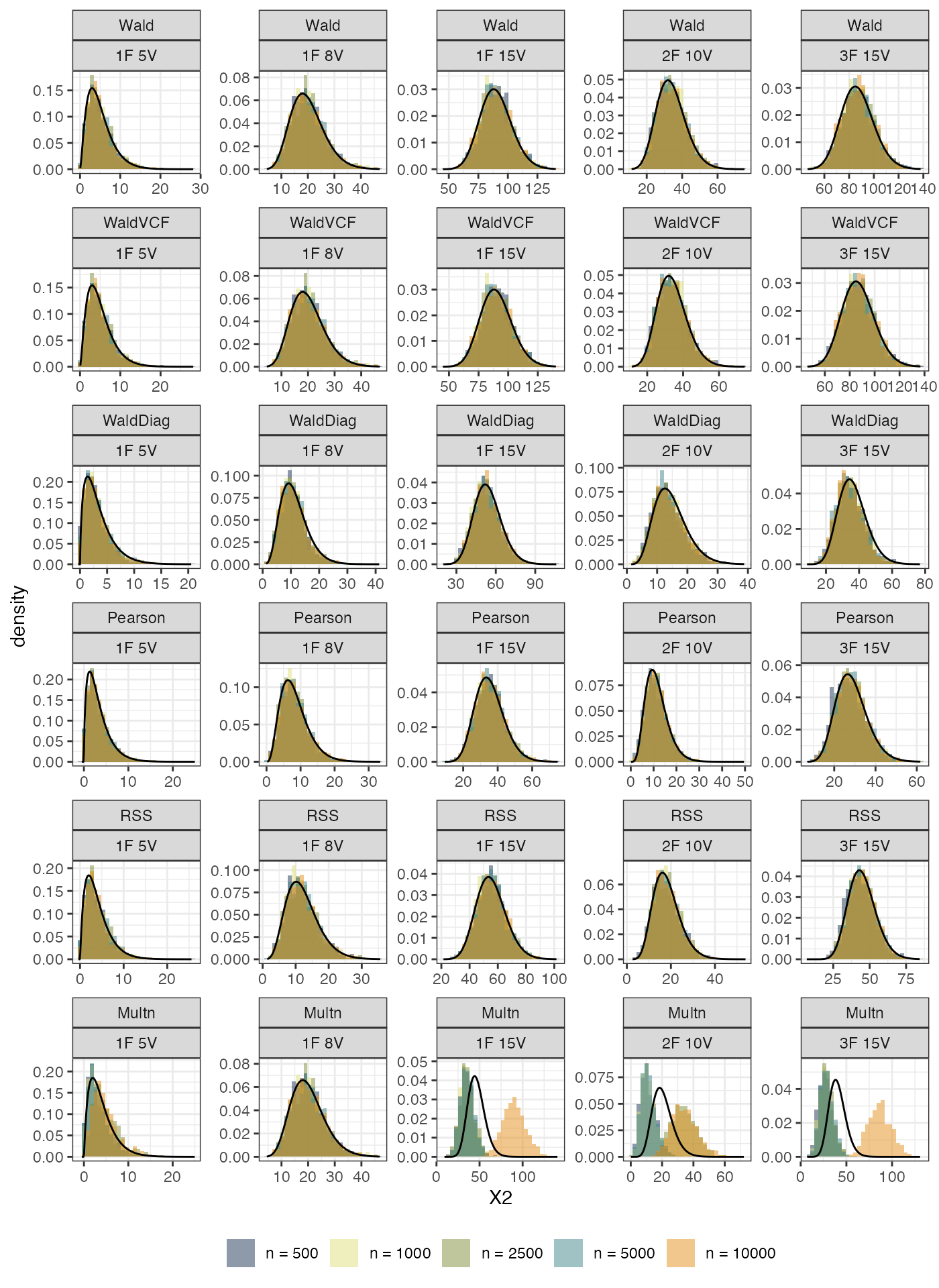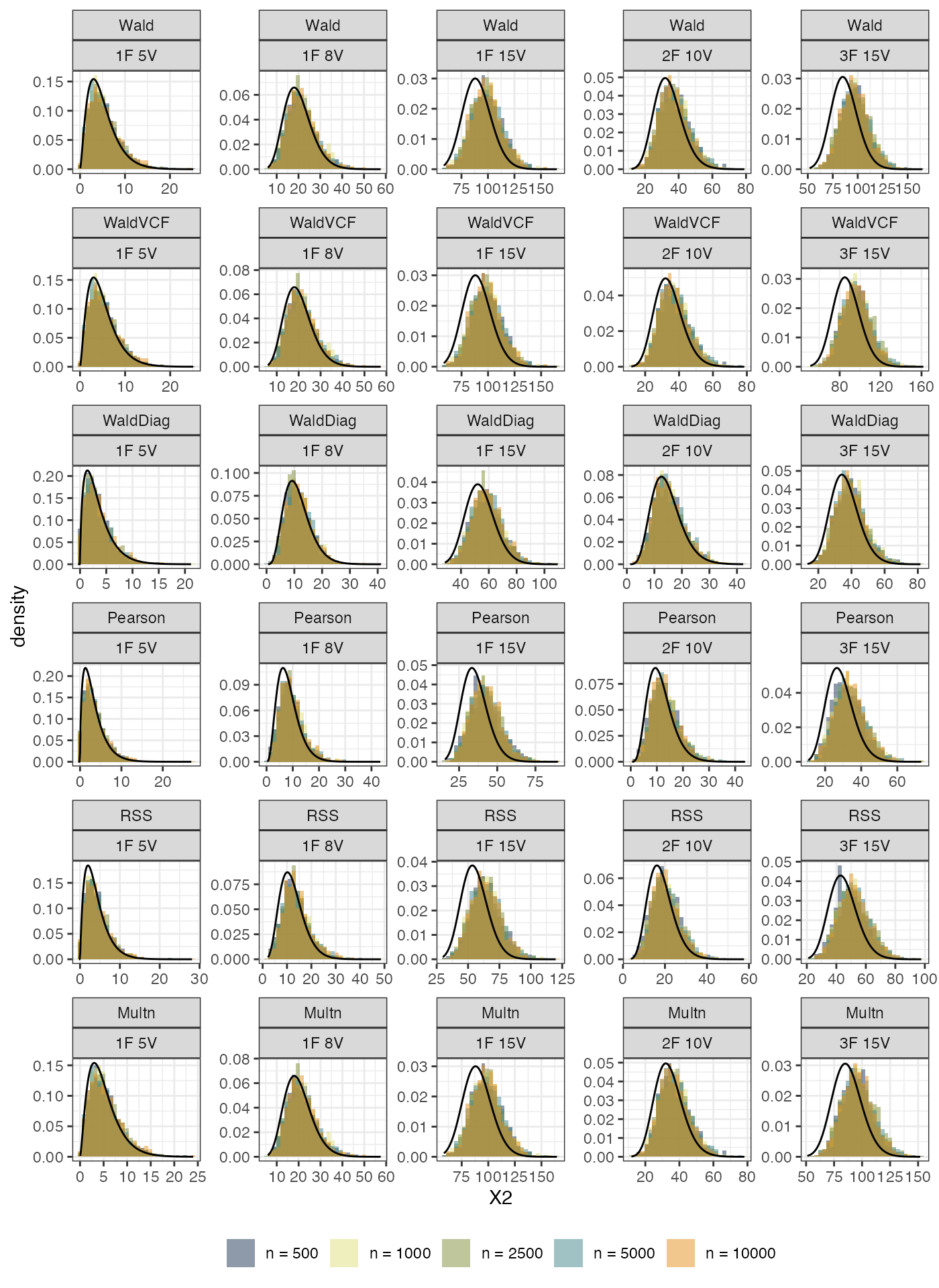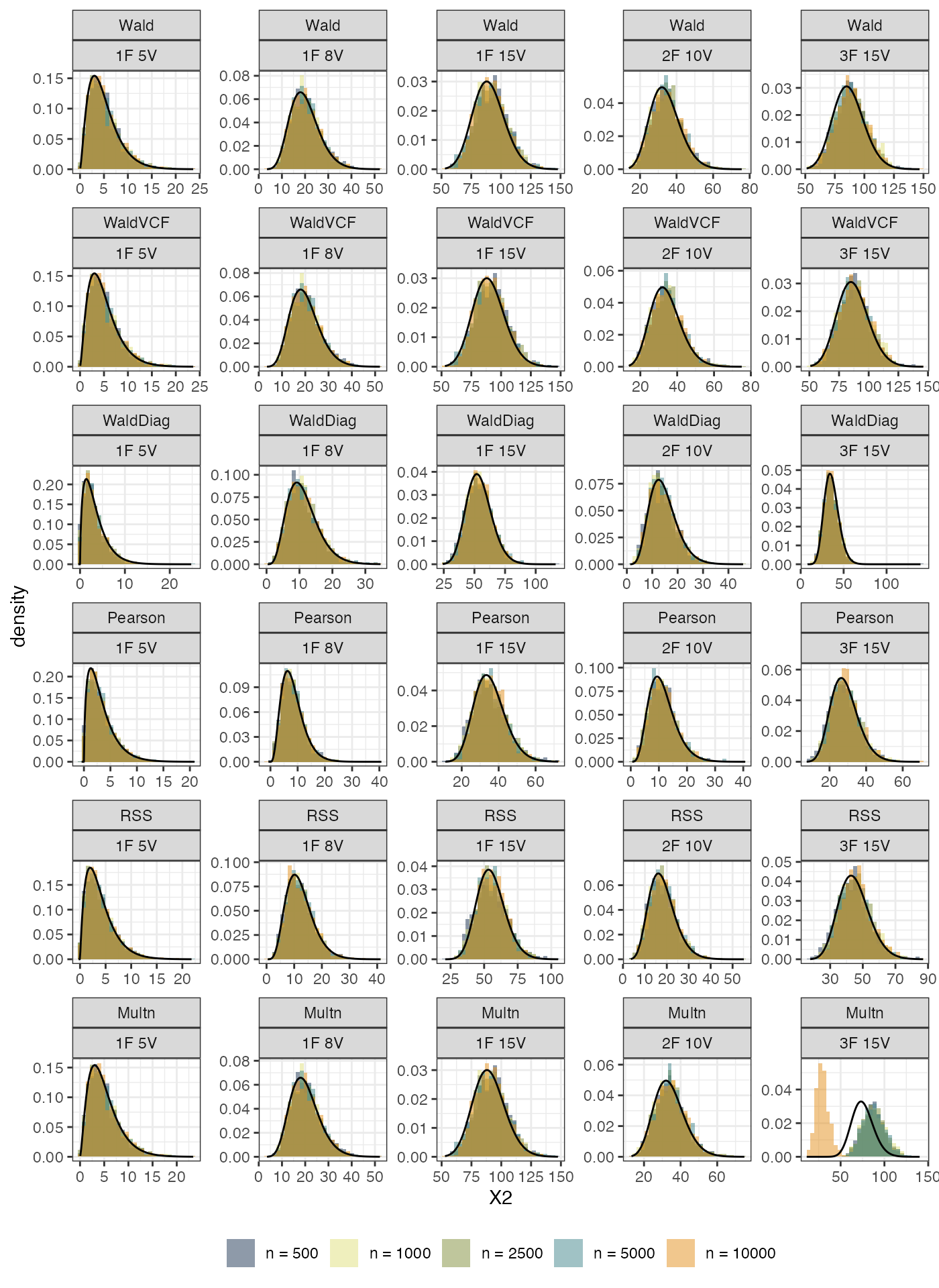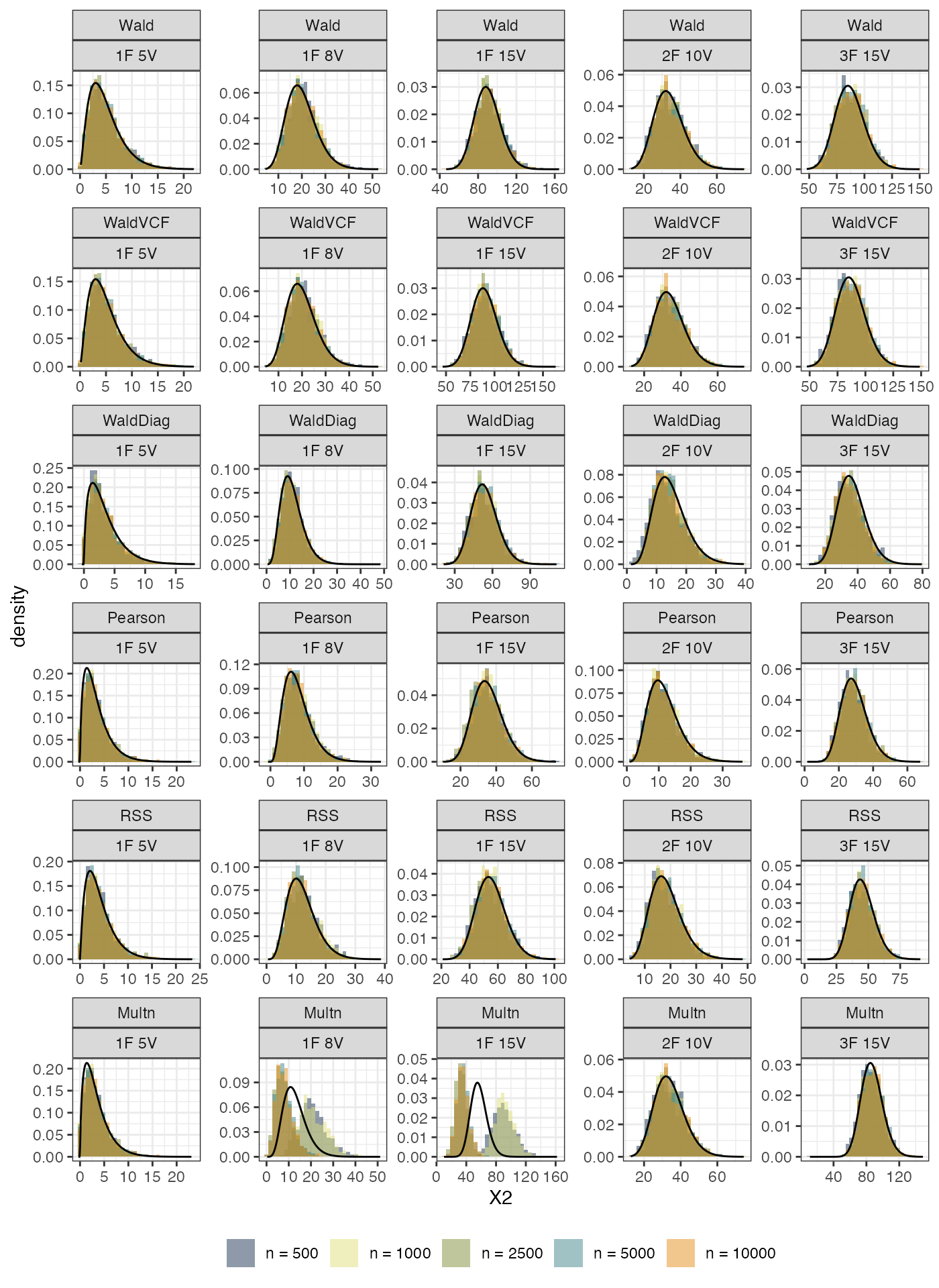A simulation study was carried out to investigate the performance of the proposed limited information goodness-of-fit tests. The data was generated using an underlying variable framework. The true parameter values were

• Loadings: $$\boldsymbol\lambda = (0.8, 0.7, 0.47, 0.38, 0.34, \dots)$$
• Factor correlations: $$\phi = 0.3$$ (1 factor) or $${\boldsymbol\phi}= (0.2, 0.3, 0.4)$$ (2 factors)
• Thresholds: $$\boldsymbol\tau = (-1.43, -0.55, -0.13, -0.82, -1.13,\dots)$$

Five scenarios were investigated:

1. 1 factor, 5 variables
2. 1 factor, 8 variables
3. 1 factor, 15 variables
4. 2 factor, 10 variables
5. 3 factor, 15 variables

For each scenario, $$B=1000$$ data were generated either according to simple random sample or a complex sampling procedure using true parameter values, and the rejection rate (Type I error) were calculated.

To conduct a power analysis, an extra latent variable $$x \sim \mathop{\mathrm{N}}(0,1)$$ independent to the latent factor $$\eta$$ was added to the $$y^*$$ variables. The loadings of $$x$$ are similar to the true values except that some noise ($$\mathop{\mathrm{N}}(0,0.1^2)$$) was added. This means that the fitted model is misspecified because a missing factor was not accounted for.

The simulations took roughly 20 hours to complete. No convergence issue reported when the sample size is in the range $$500 \leq n \leq 3000$$ (previous versions of this simulation study saw lavaan having difficulty converging when $$n=100$$ or $$n=250$$–these simulation results are not trustworthy so have been omitted).

## Path diagrams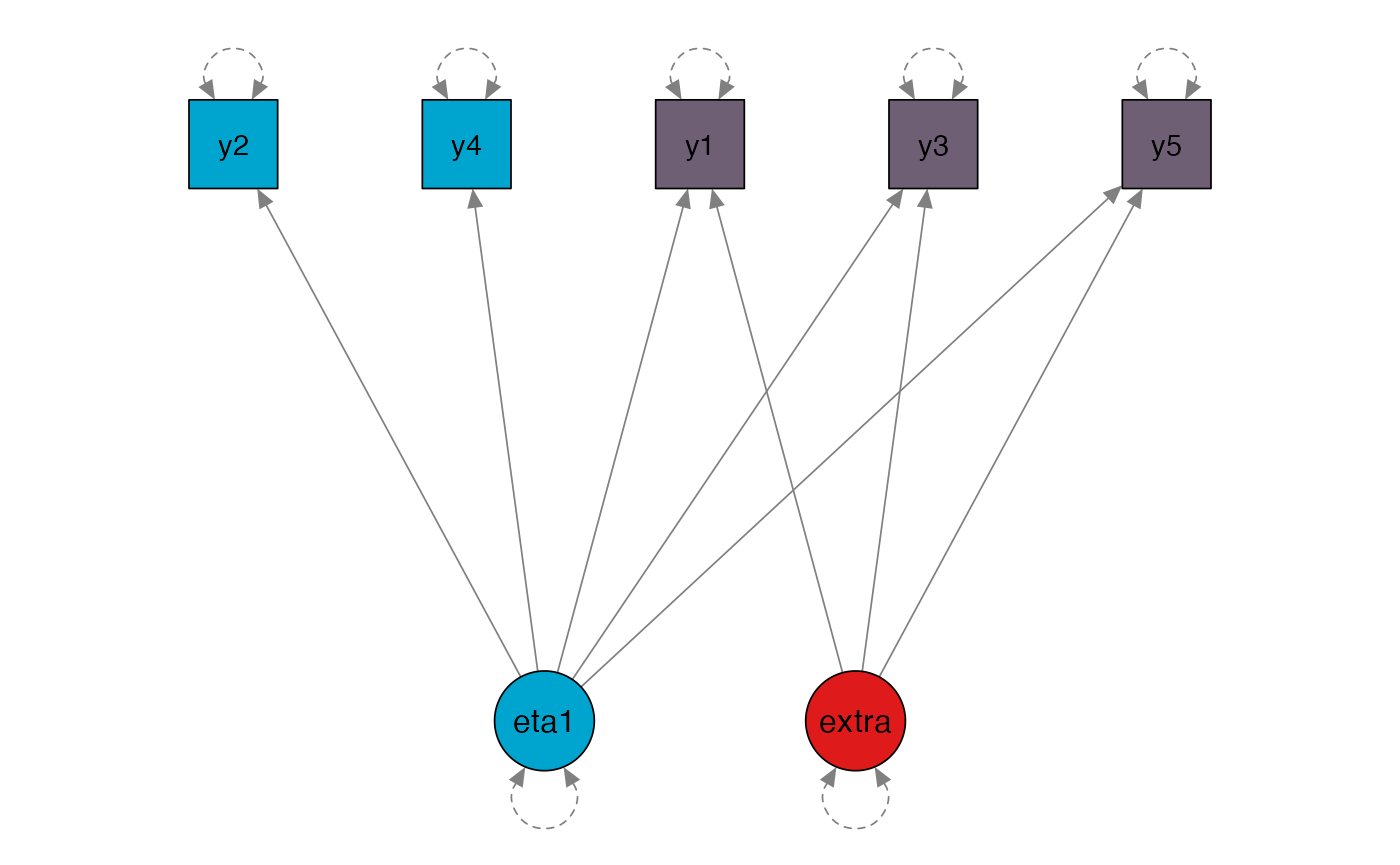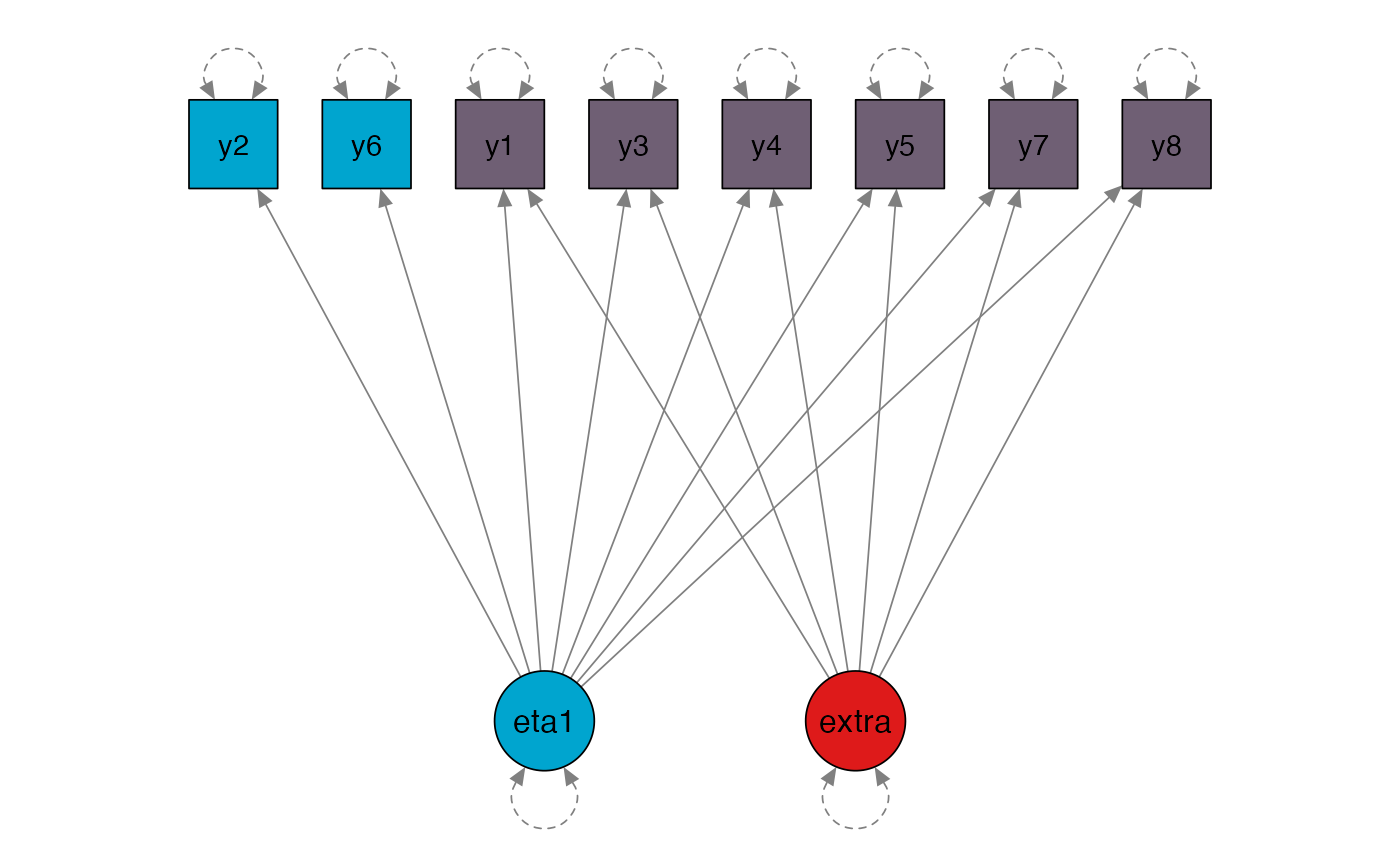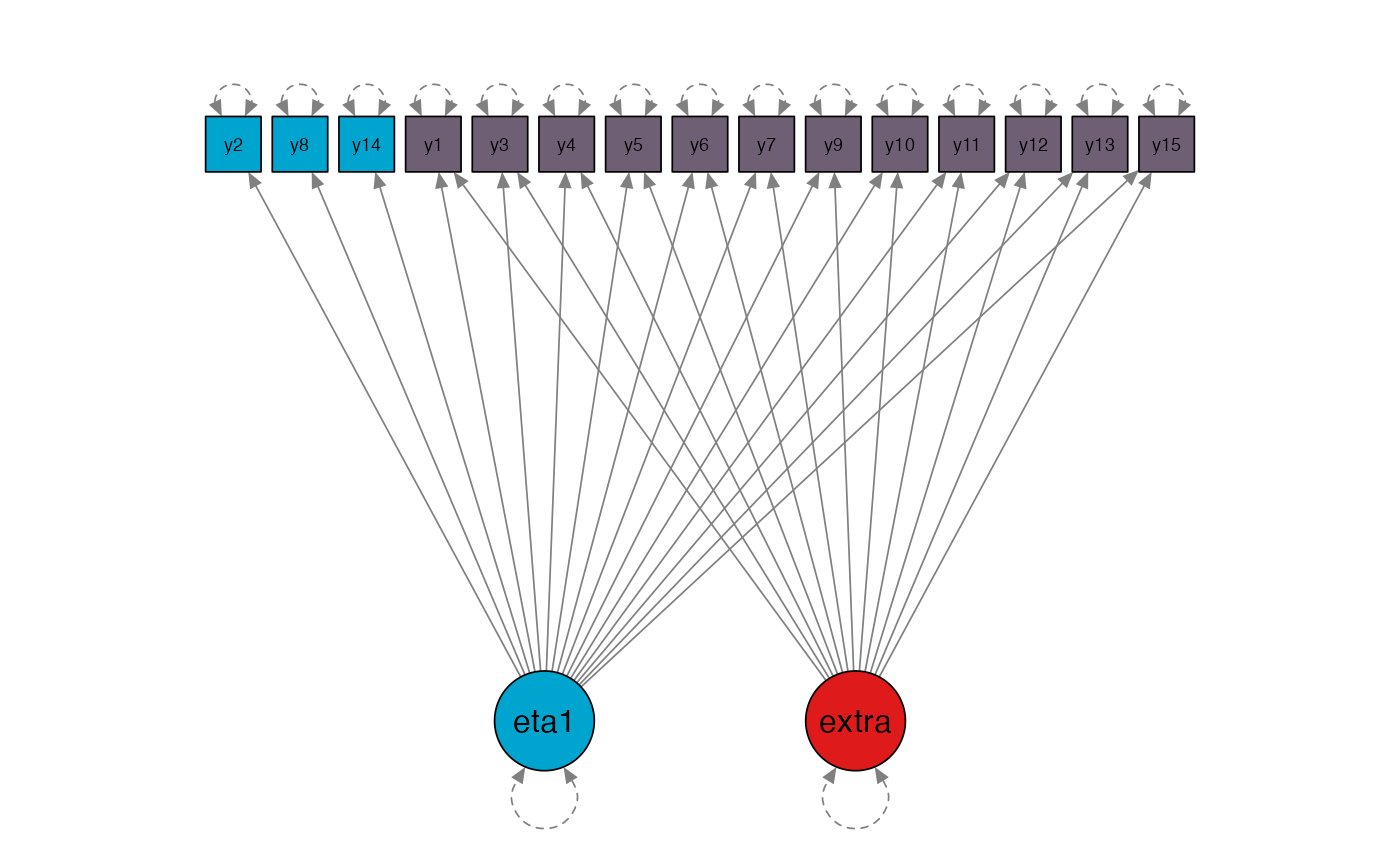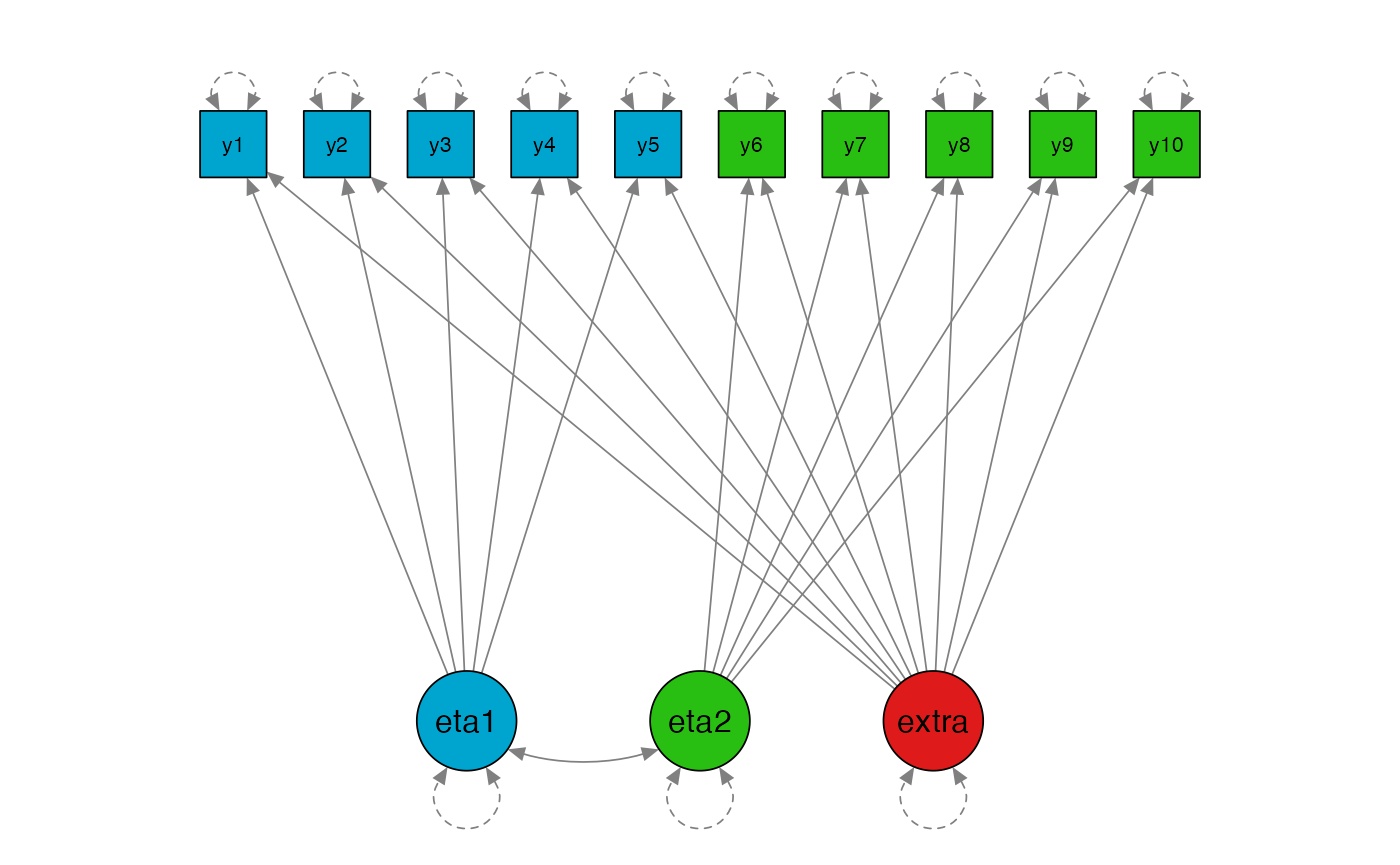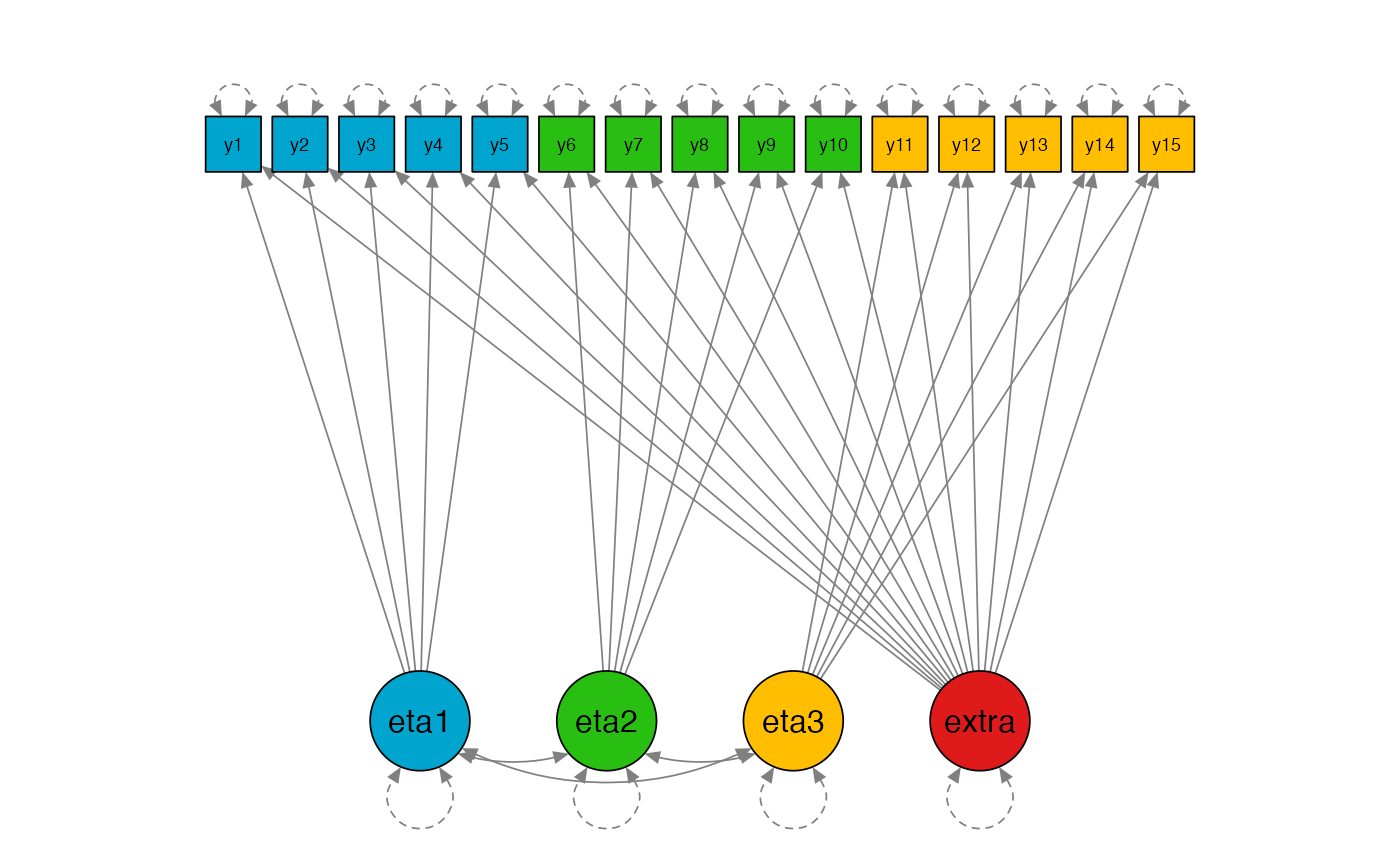## SRS type I errors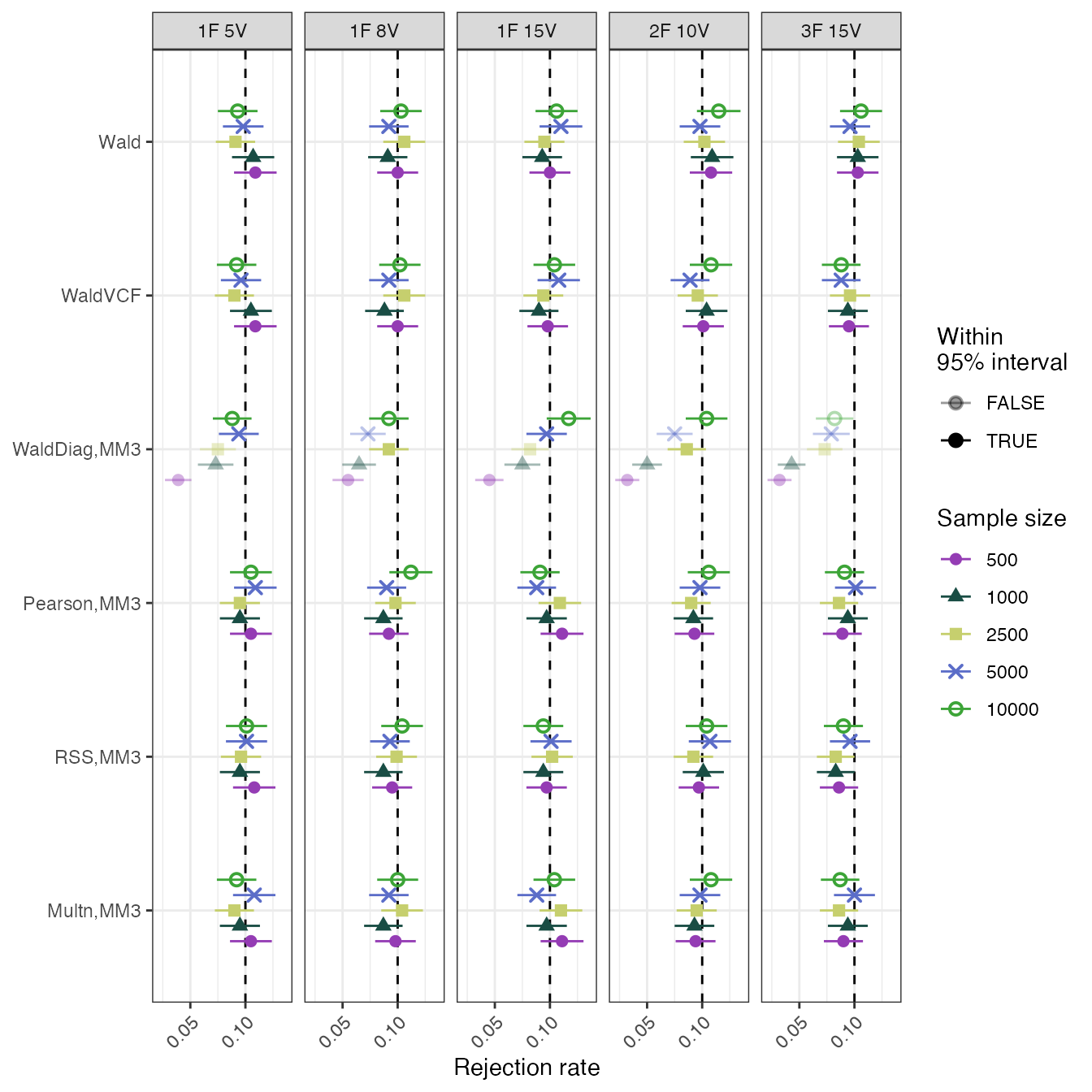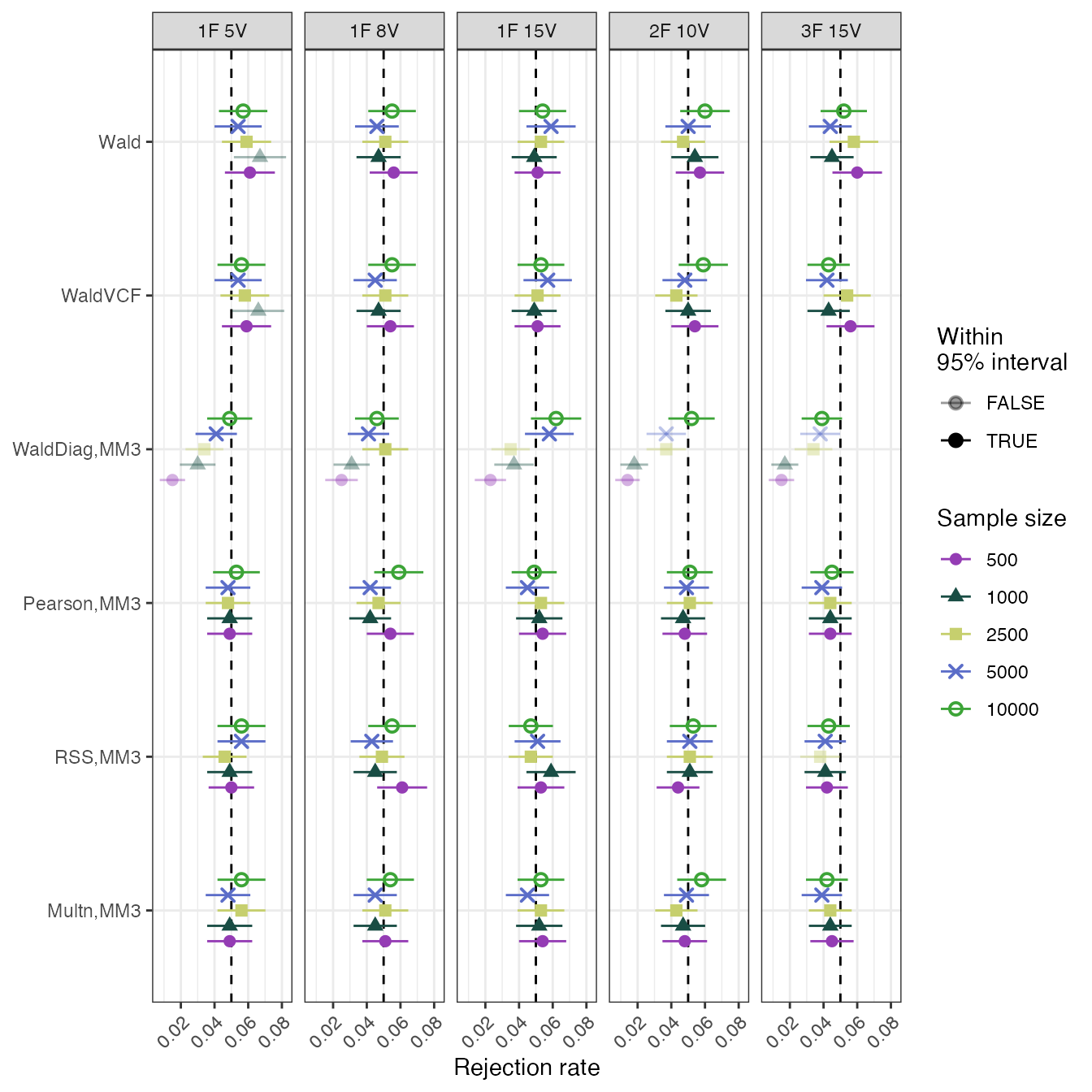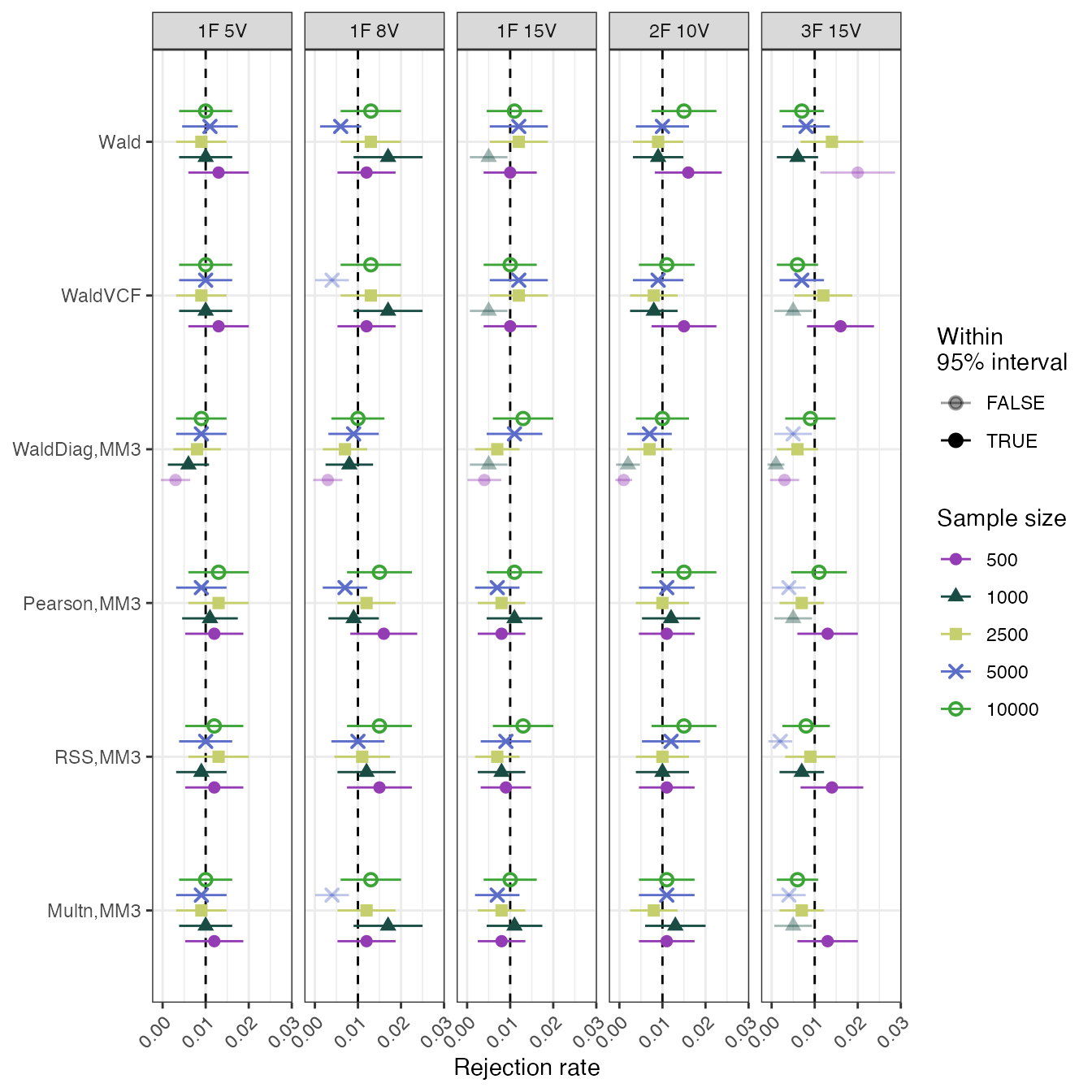## SRS power plots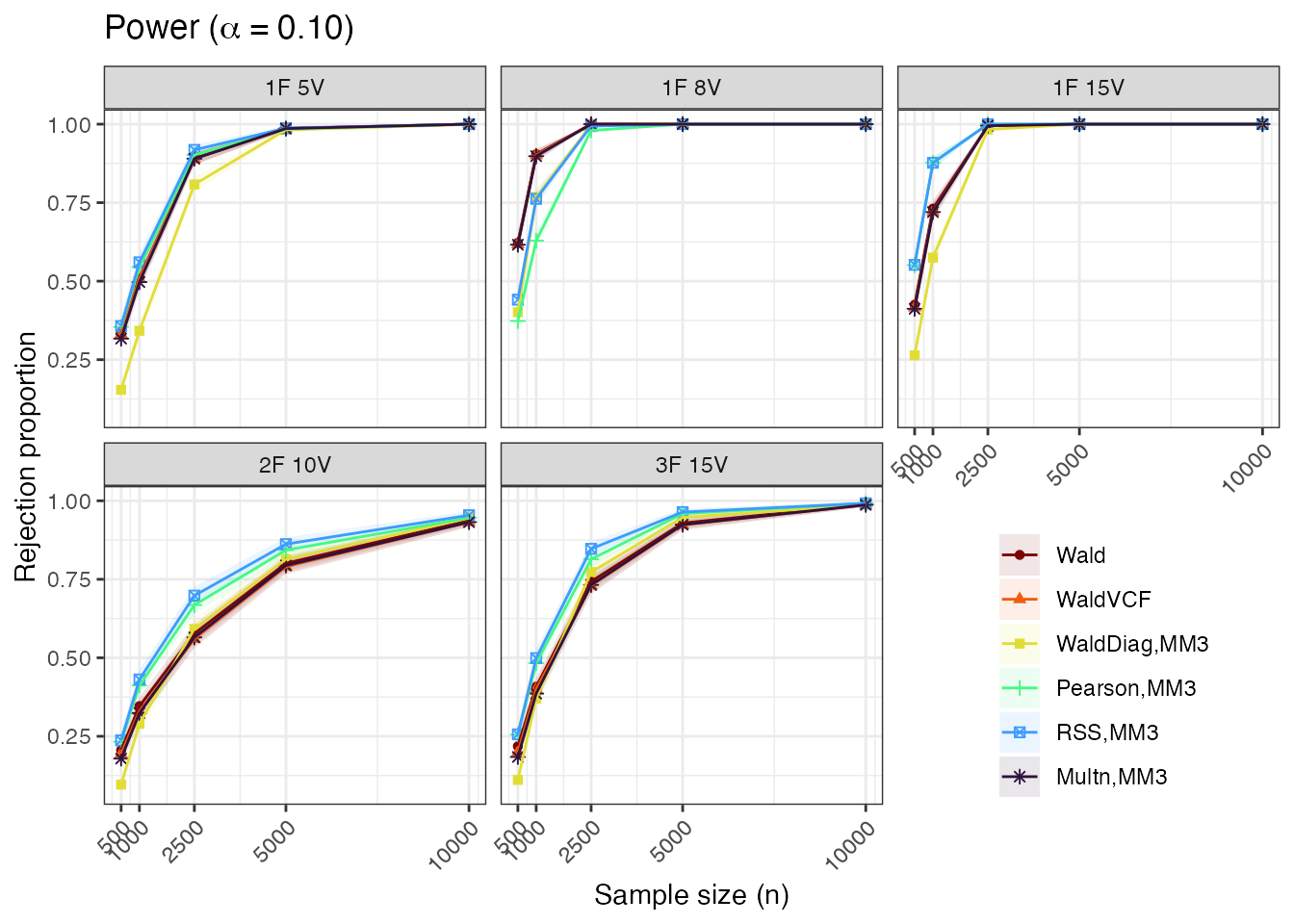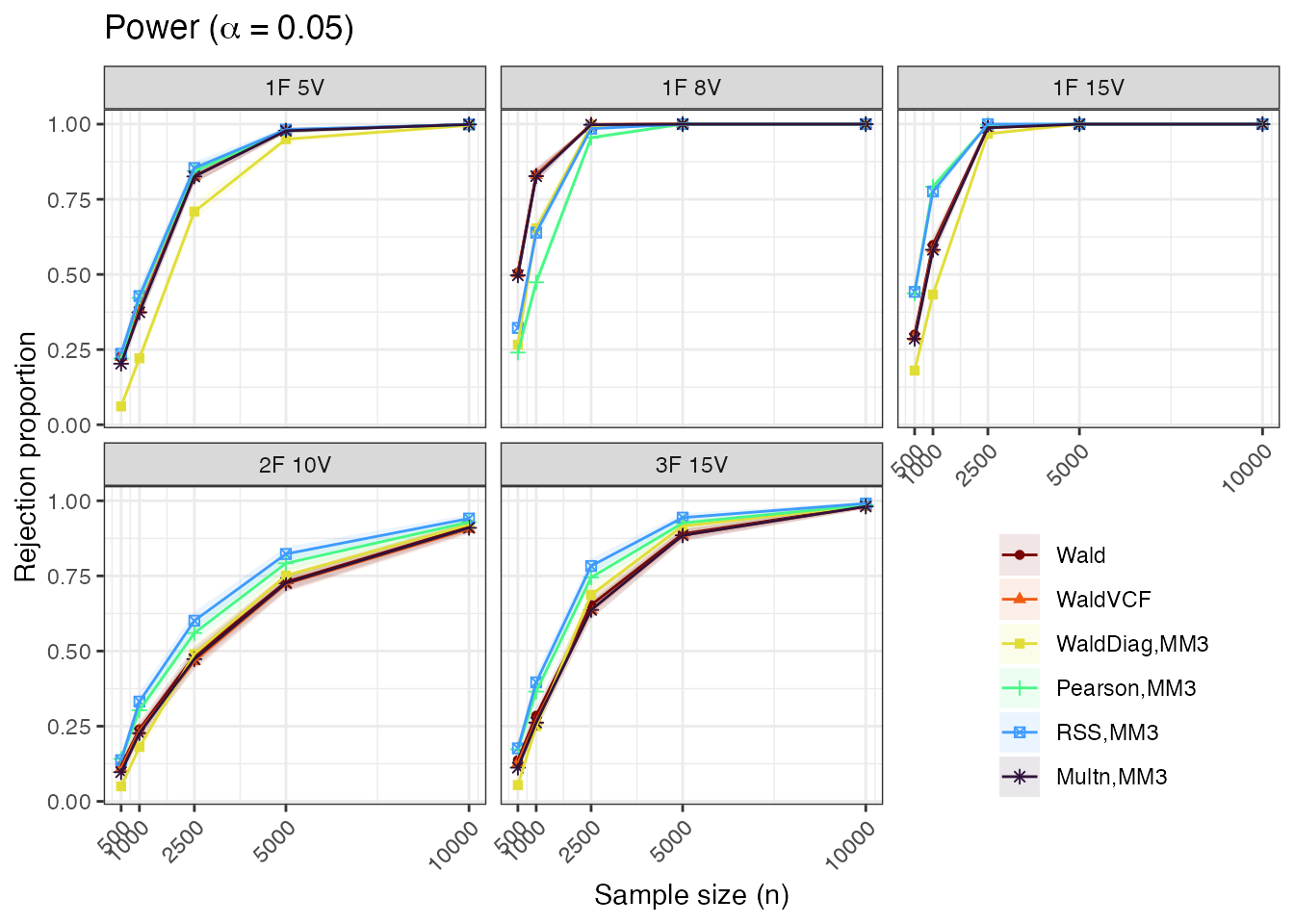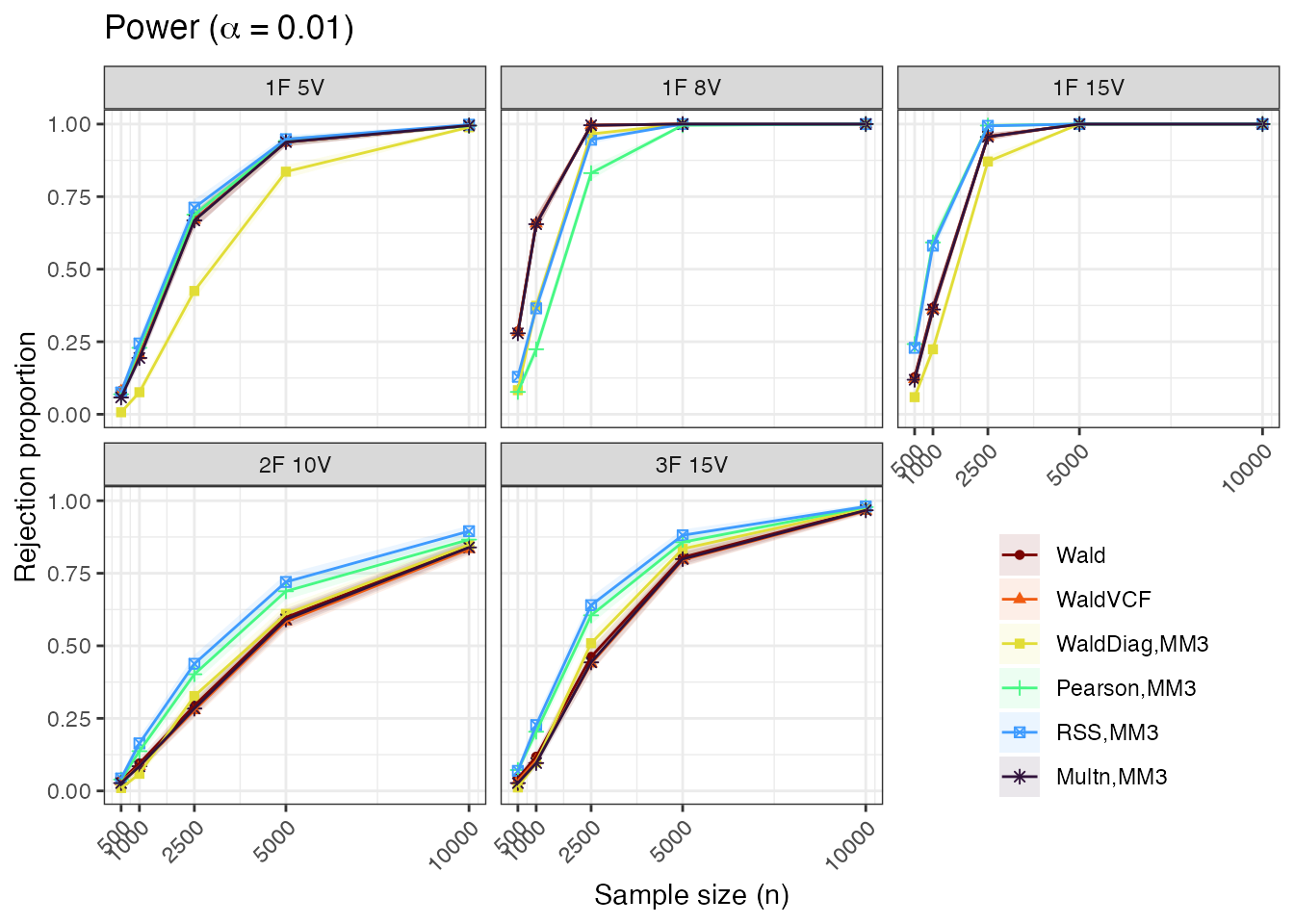## Complex sampling type I errors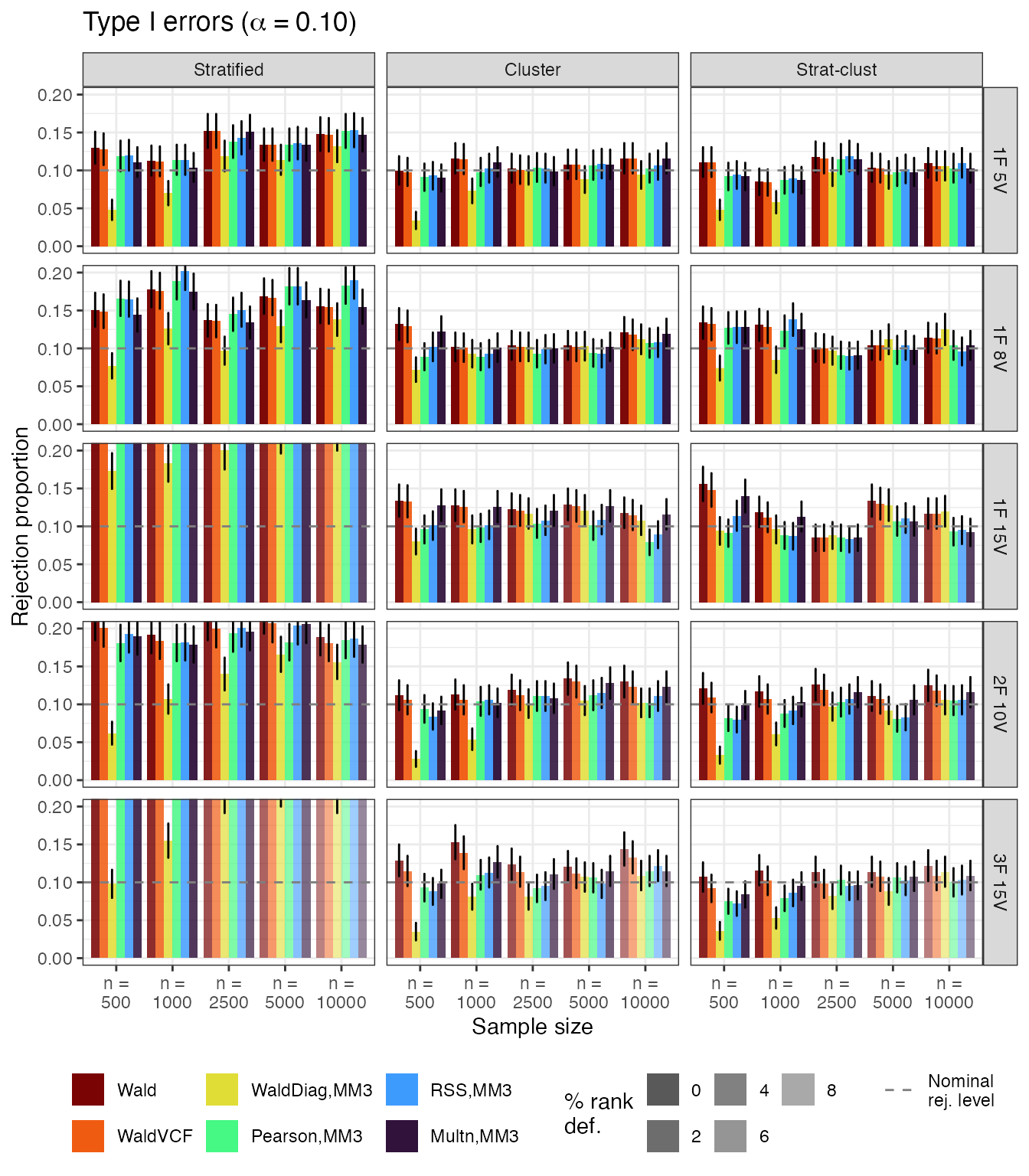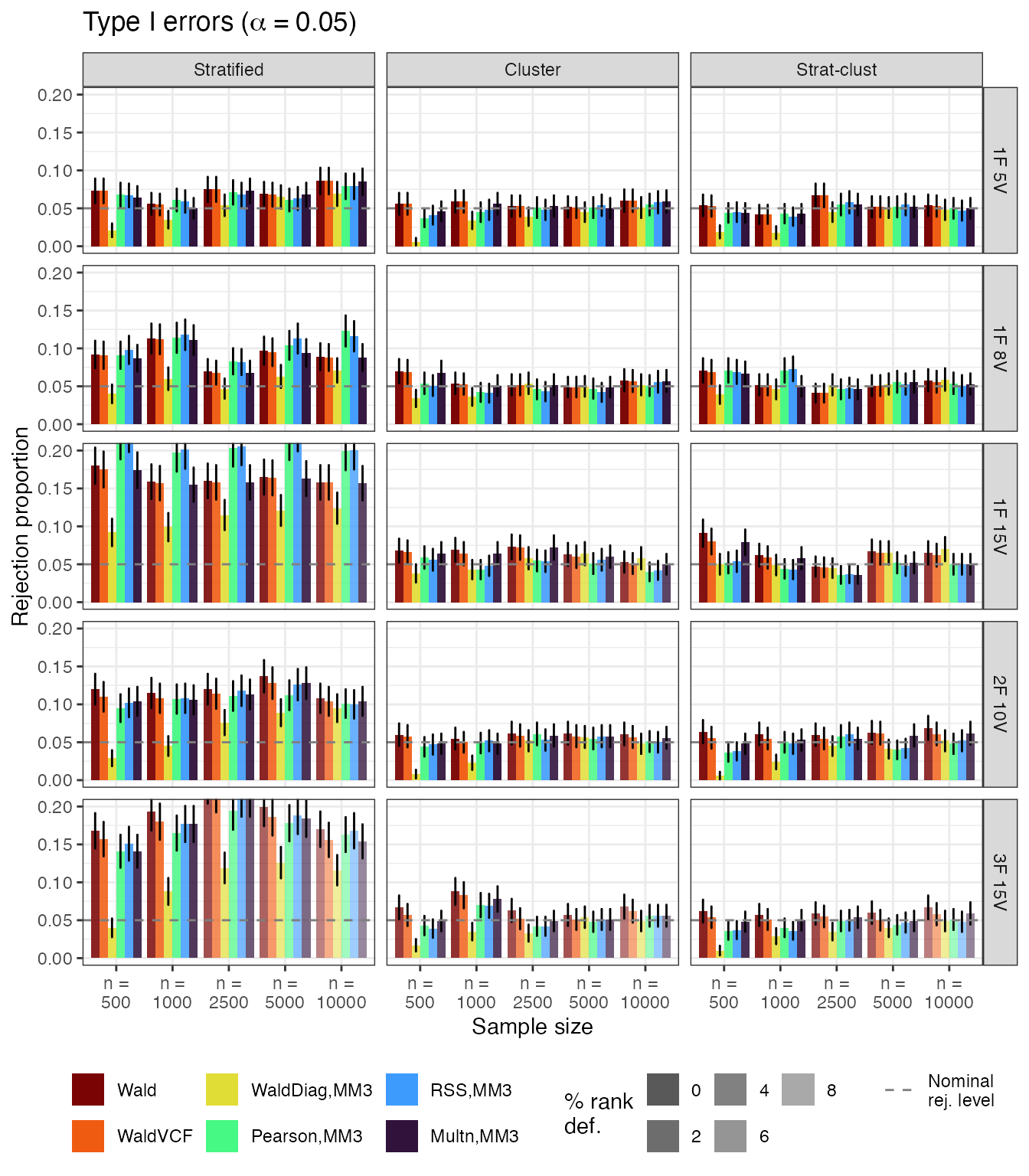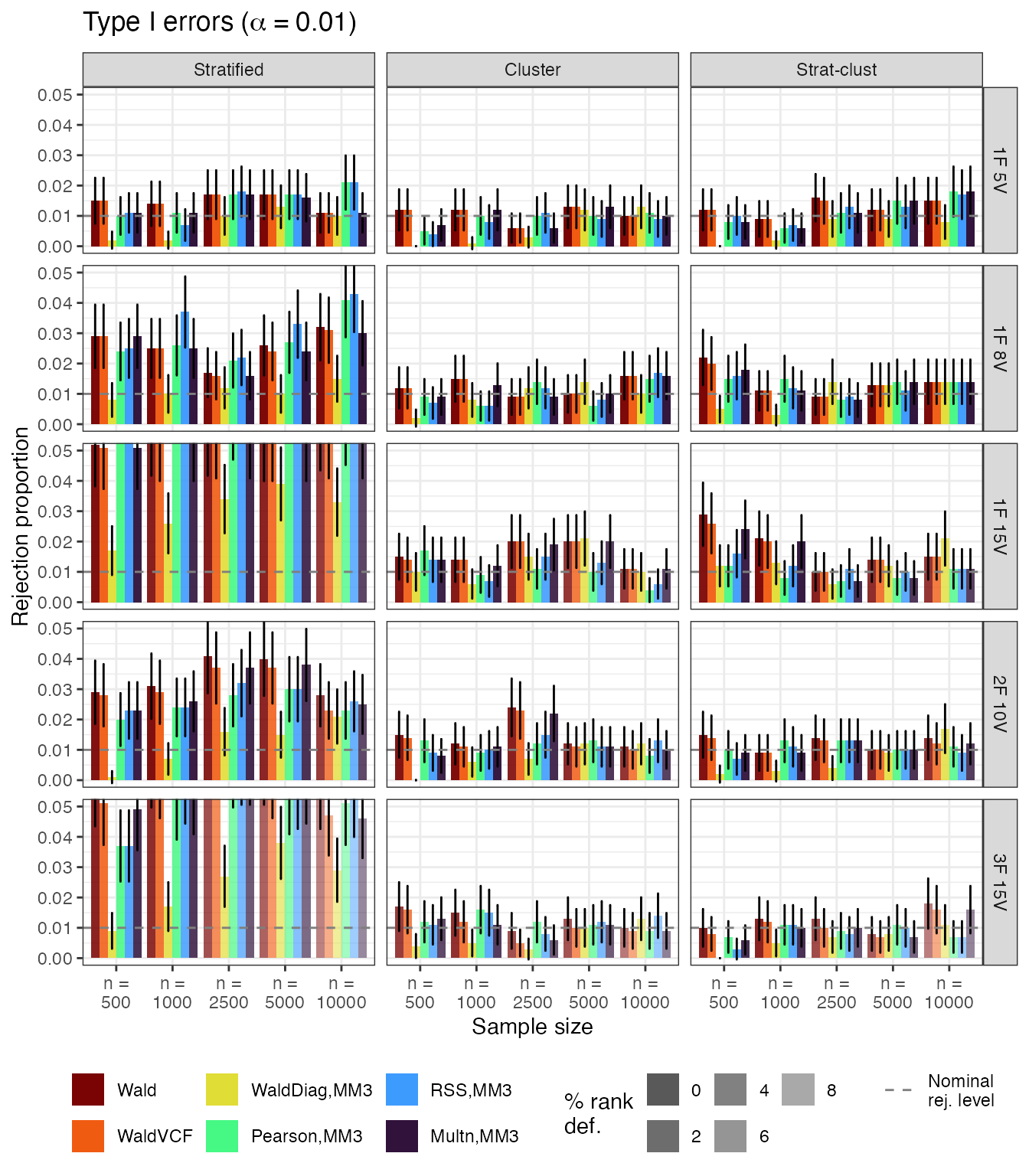## Complex sampling power plots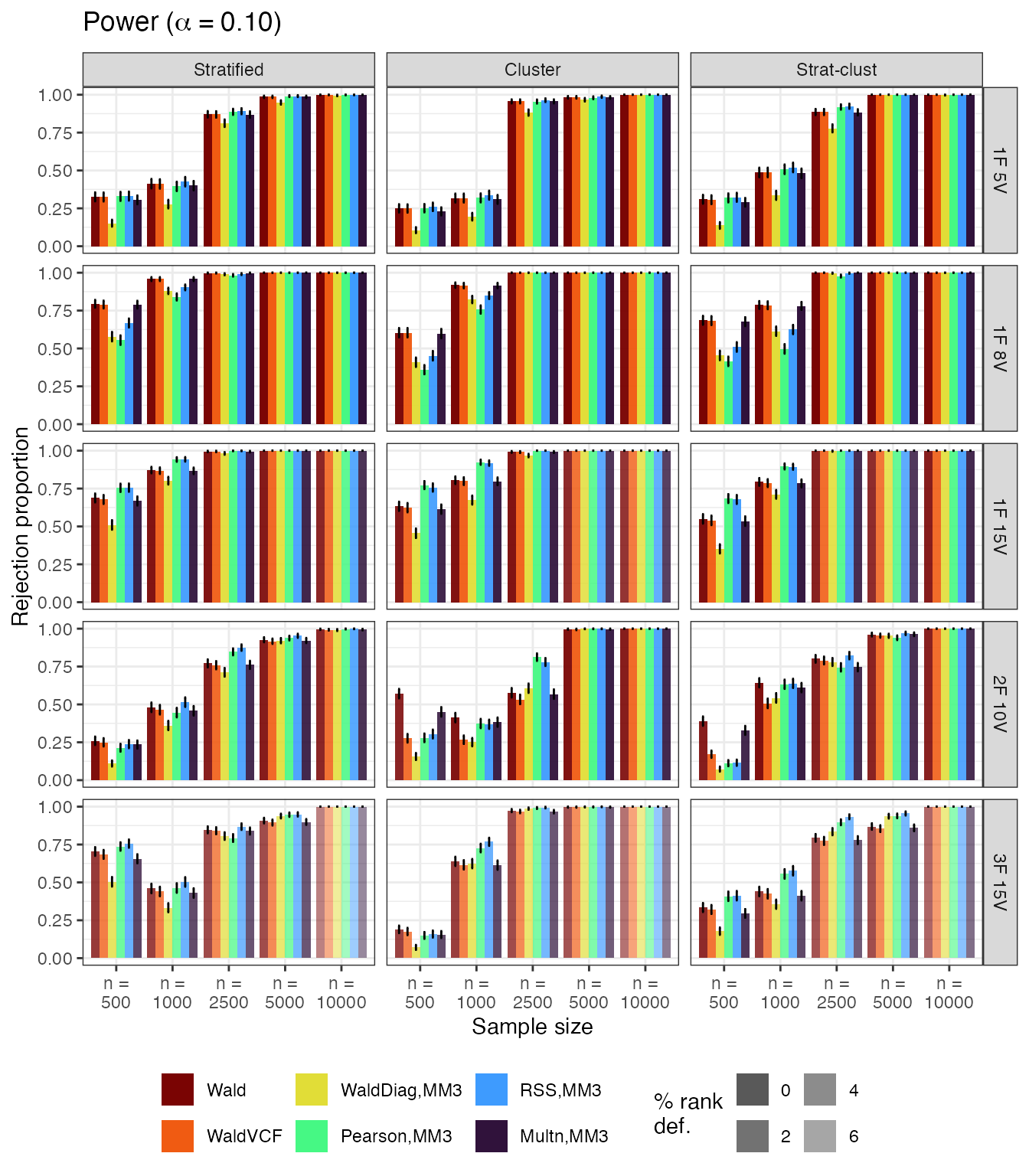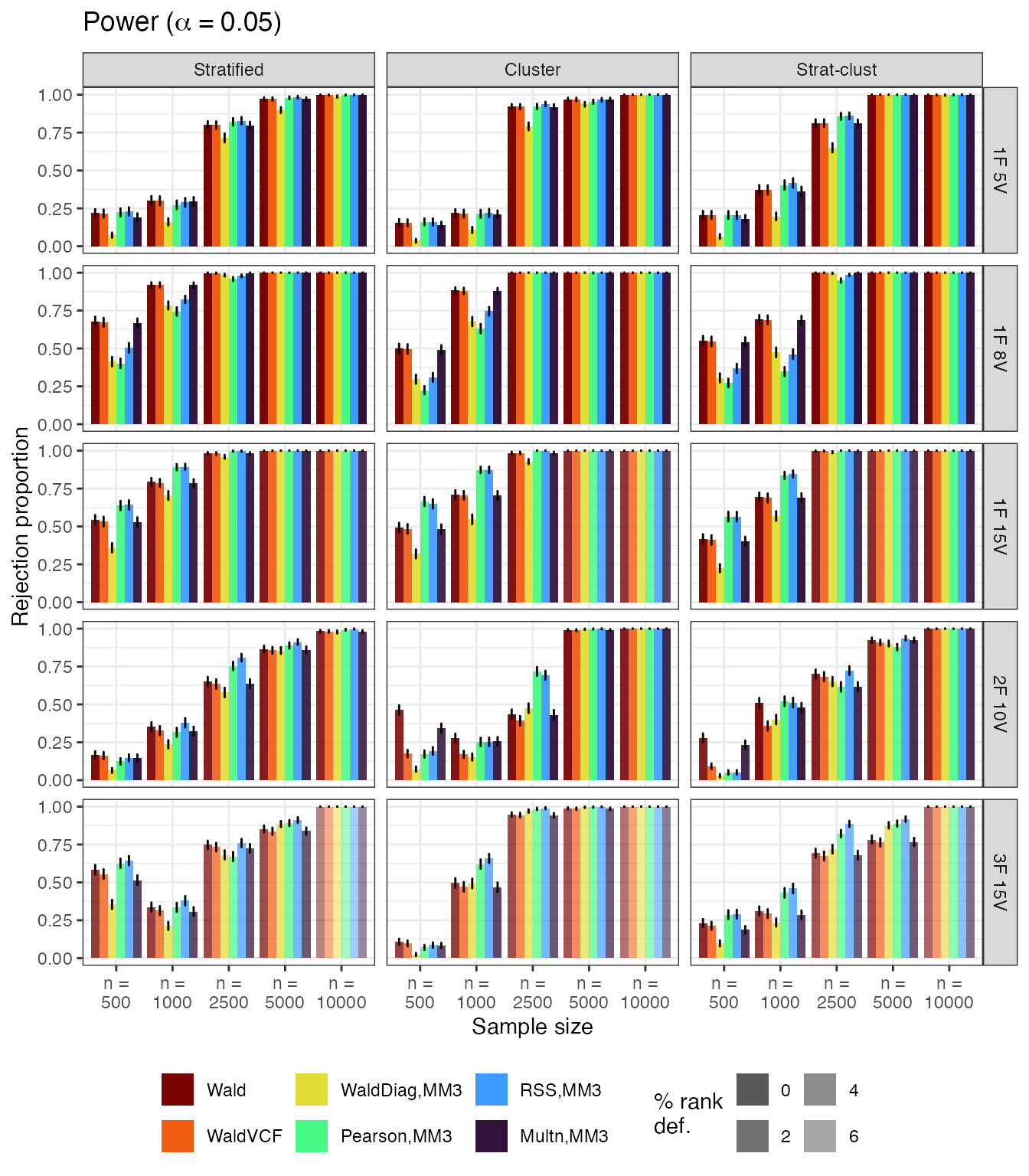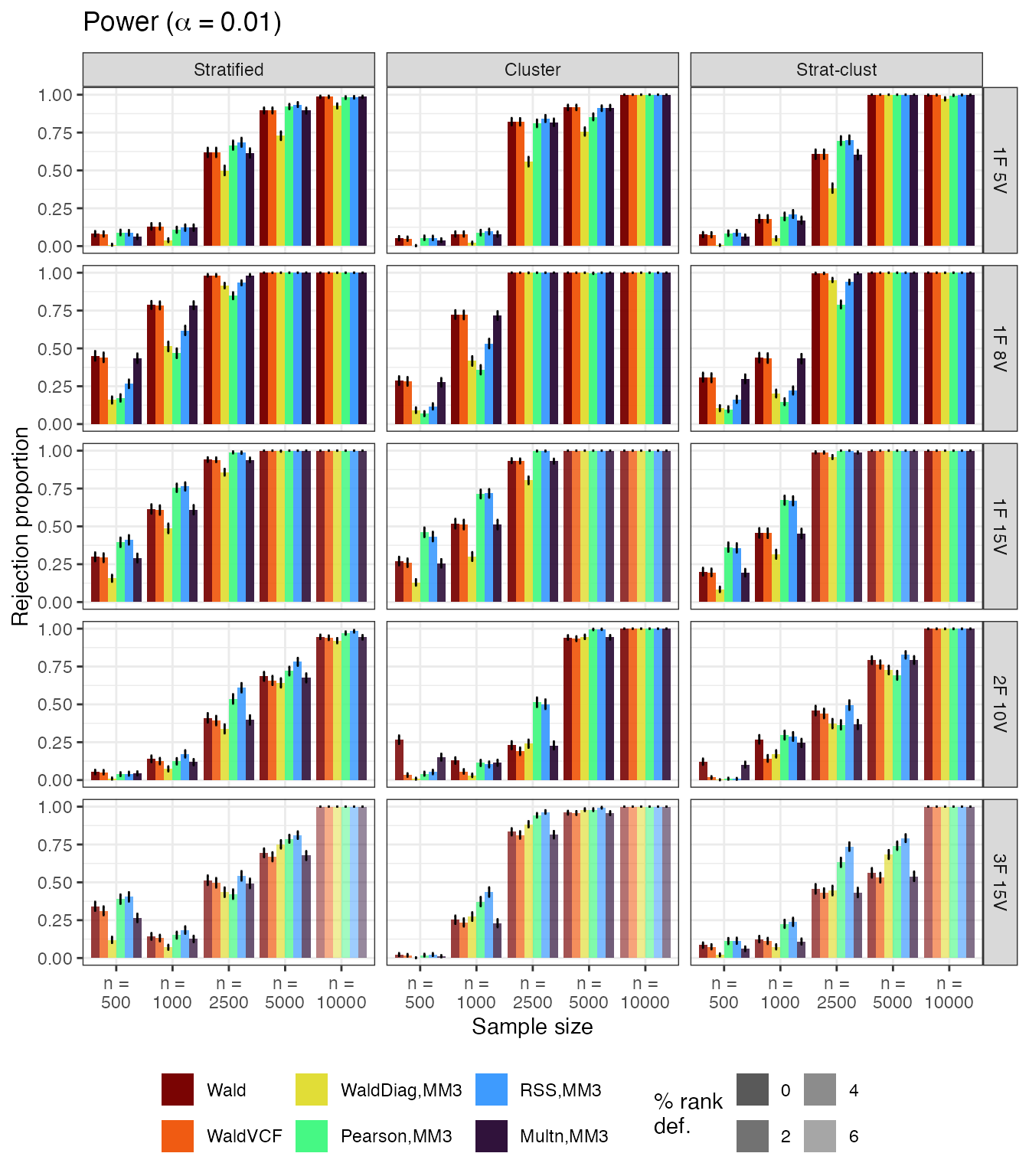## Distribution of test statistics